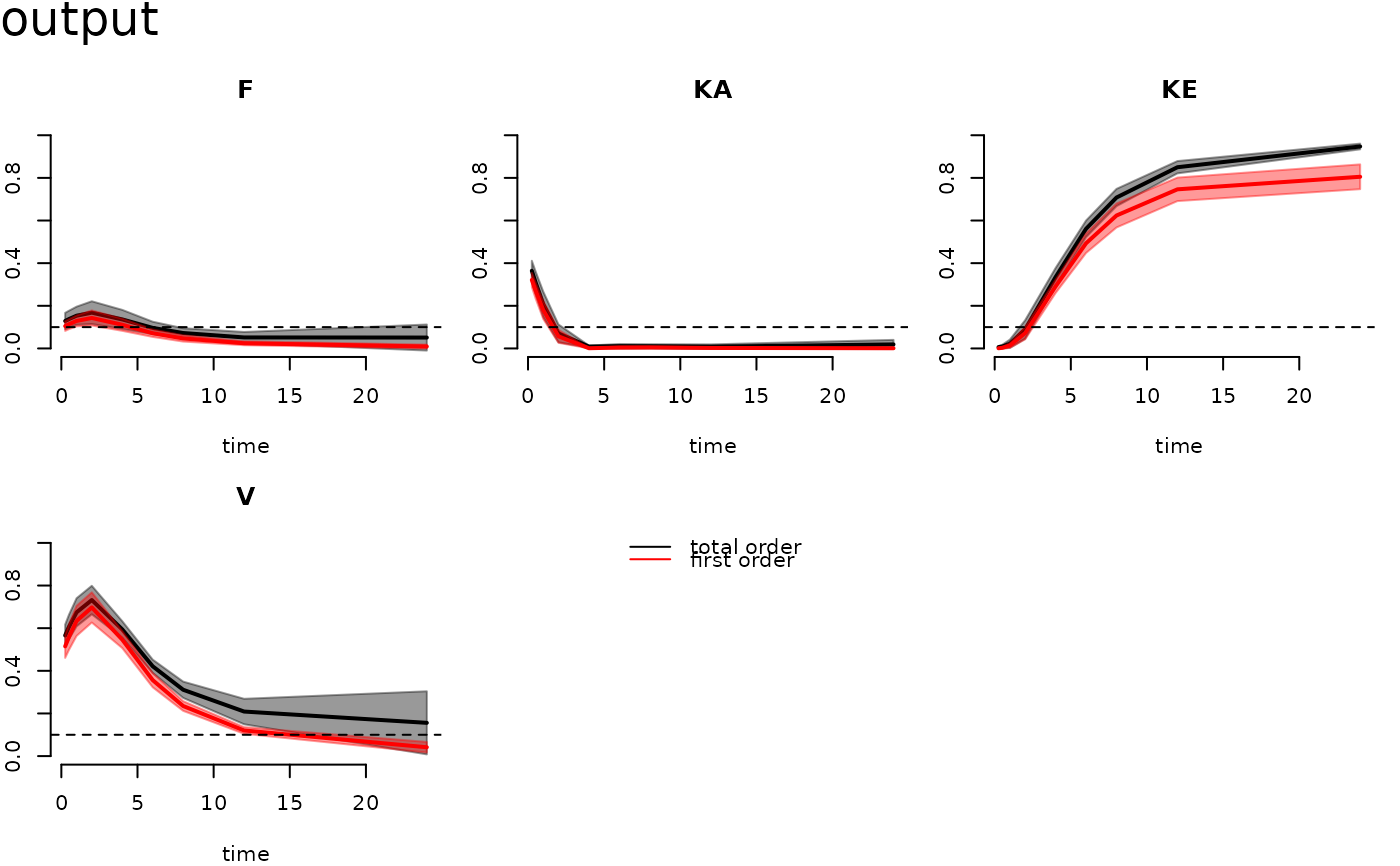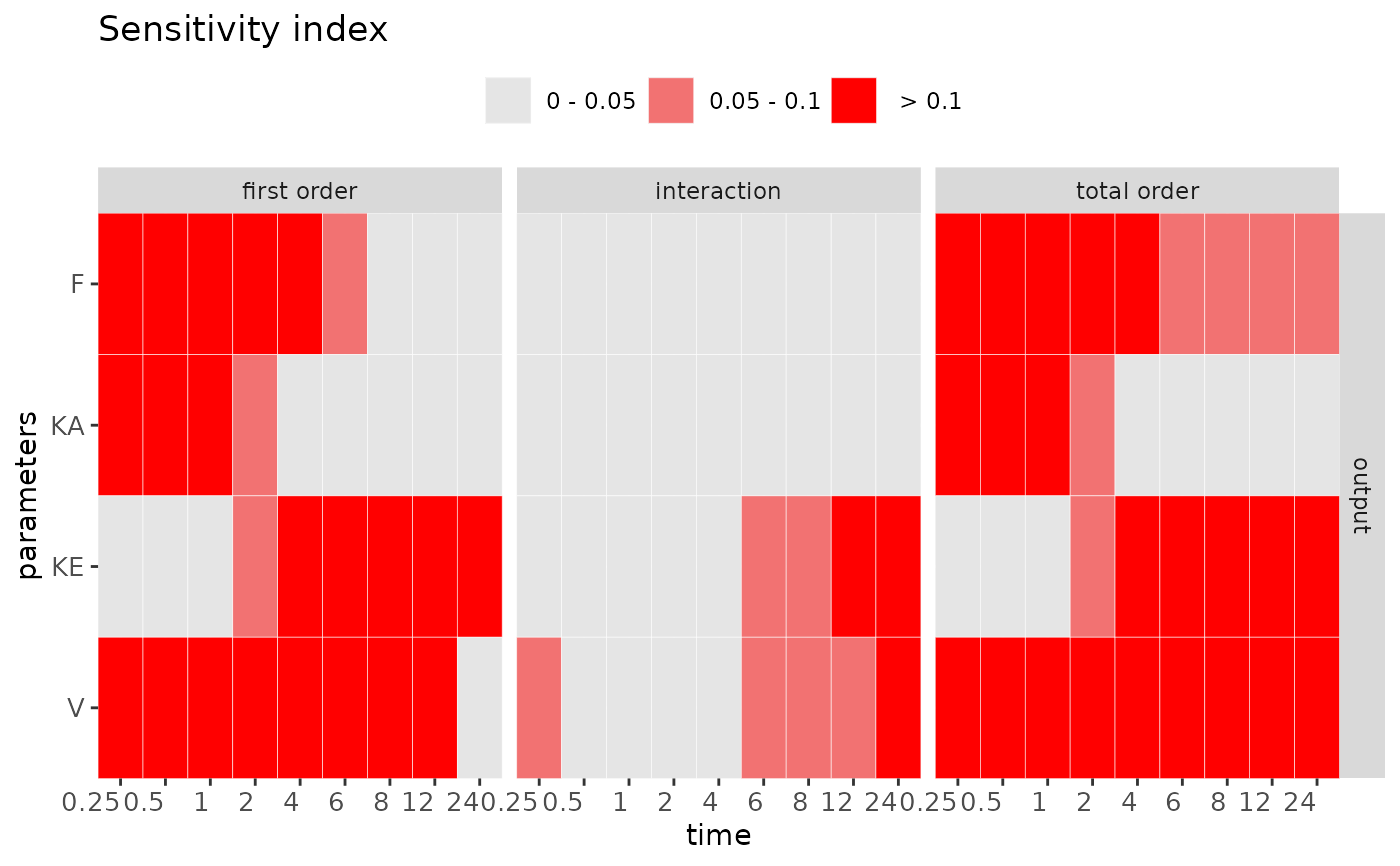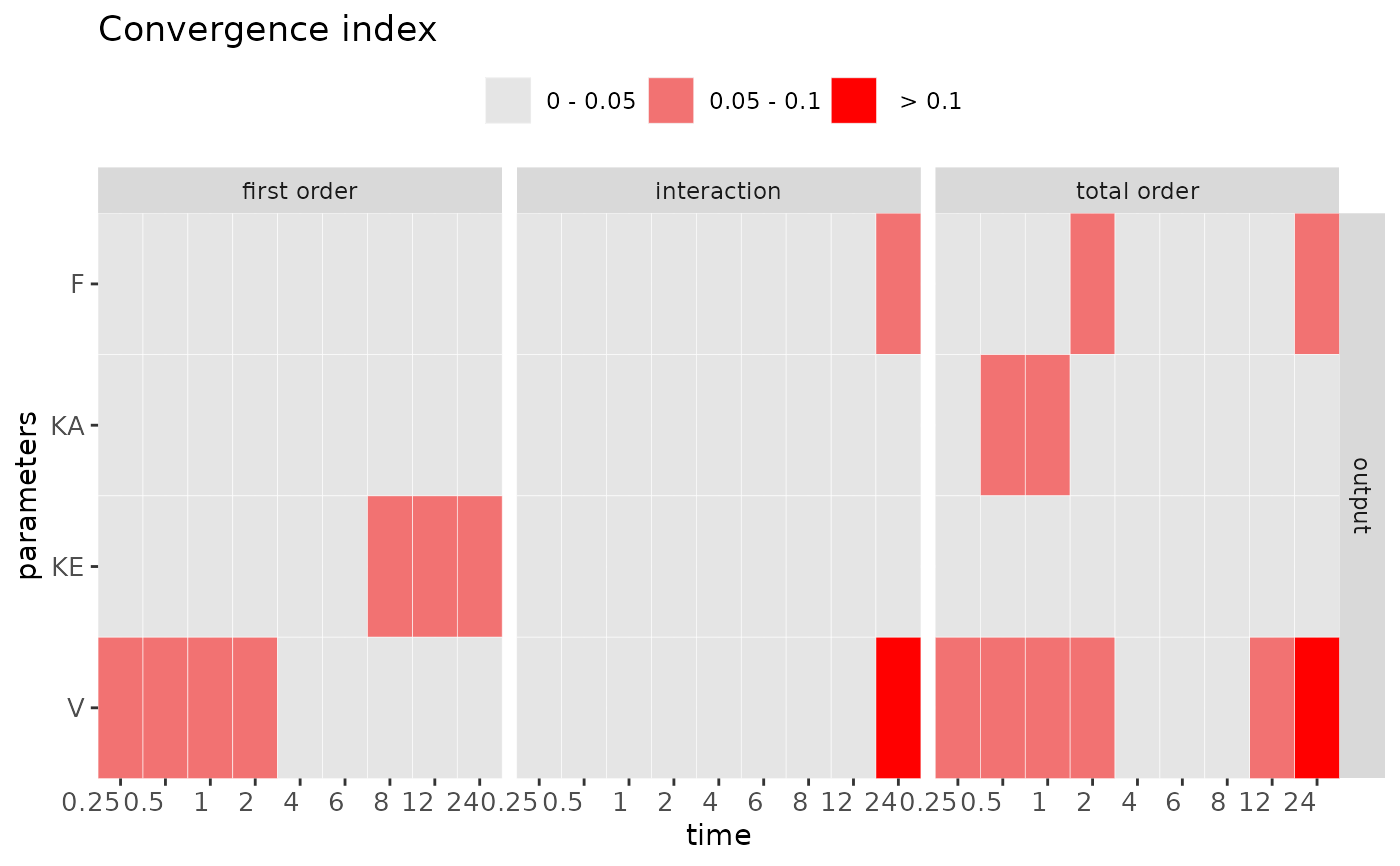Visualize and check the sensitivity (or convergence) measurement with the given result.

check(x, times, vars, SI.cutoff, CI.cutoff, out)

heat_check(
x,
order = c("first order", "interaction", "total order"),
vars = NULL,
times = NULL,
SI.cutoff = c(0.05, 0.1),
CI.cutoff = c(0.05, 0.1),
index = "SI",
level = T,
text = F,
show.all = FALSE
)

# S3 method for rfast99
plot(x, vars = 1, SI.cutoff = 0.1, ...)

# S3 method for rfast99
print(x, ...)

## Arguments

x a list of the storing information in the defined sensitivity function. a logical value or character to specify the display time in simulation. a logical value or character to specify the display variable in simulation. a value or vector to set the cut-off for sensitivity index. The default is 0.05. a value or vector to set the cut-off for convergence index. The default is 0.05. a logical value to print the checking result to the console. a vector of the interested output index, including first order, interaction, and total order. a character to choose sensitivity index SI (default) or convergence index CI. a logical value to use continuous or discrete (default) output. a logical value to display the calculated indices in the plot. a logical value to show all testing parameters in the heatmap. The default is set to FALSE to show only the influential parameters. additional arguments to customize the graphical parameters.

## Value

The print function returns sensitivity and convergence indices with given time-step in the console. The check method provides the summary of parameter sensitivity and convergence according to the given SI.cutoff and CI.cutoff. It can distinguish the influential and non-influential parameter by the providing value of SI.cutoff. The plot function can generate the time-course functional outputs of first order and interaction indices for each parameter. The default output is the first model variable. The heat_check provides a convenient way to visualize and distinguish the influential and non-influential parameter by the setting cut-off. The convergence index can examine the stability of the sensitivity index. To check convergence, be sure to conduct the replication in rfast99.

## Details

The convergence of sensitivity indices for each parameter is using the approach proposed by Sarrazin et al. (2016). This method quantitatively assesses the convergence by computing the range of 95\ Using the global approach based on the heatmap visualization combined with the index "cut-off," can systematically distinguish between "influential" and "non-influential" parameters (Hsieh et al. 2018).

Sarrazin, F., Pianosi, F., & Wagener, T. (2016). Global Sensitivity Analysis of environmental models: Convergence and validation. Environmental Modelling & Software, 79, 135–152.

Hsieh, N. H., Reisfeld, B., Bois, F. Y., & Chiu, W. A. (2018). Applying a global sensitivity analysis workflow to improve the computational efficiencies in physiologically-based pharmacokinetic modeling. Frontiers in Pharmacology, 9, 588.

tell2

## Examples

q <- "qunif"
q.arg <- list(list(min = 0.6, max = 1),
list(min = 0.5, max = 1.5),
list(min = 0.02, max = 0.3),
list(min = 20, max = 60))

params <- c("F","KA","KE","V")

set.seed(1234)
x <- rfast99(params = params, n = 200, q = q, q.arg = q.arg, rep = 20)

time <- seq(from = 0.25, to = 12.25, by = 0.5)
out <- solve_fun(x, model = FFPK, time = time, vars = "output")
#> Starting time: 2021-07-07 11:07:42#> Ending time: 2021-07-07 11:07:43

# Check results of sensitivity measures
check(out)
#>
#> Sensitivity check ( Index > 0.05 )
#> ----------------------------------
#> First order:
#>  F KA KE V
#>
#> Interaction:
#>  KE V
#>
#> Total order:
#>  F KA KE V
#>
#> Unselected factors in total order:
#>
#>
#>
#> Convergence check ( Index > 0.05 )
#> ----------------------------------
#> First order:
#>  KE V
#>
#> Interaction:
#>
#>
#> Total order:
#>  F KA V
#> plot(out)heat_check(out, show.all = TRUE)heat_check(out, index = "CI")